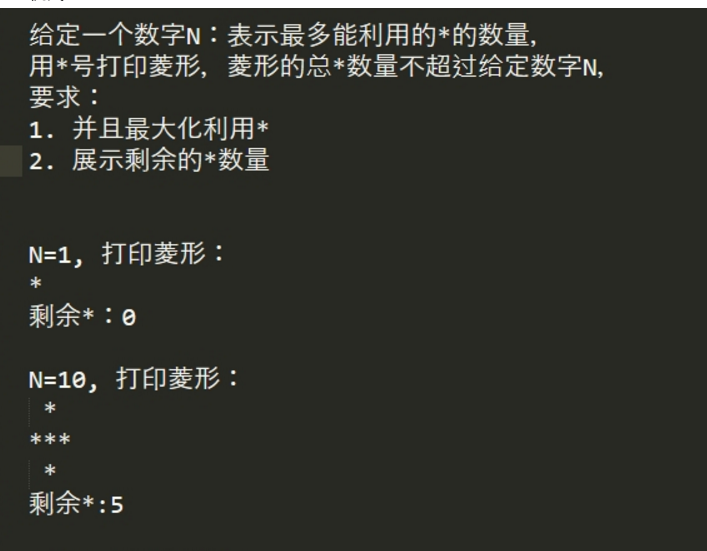2021-02-19 12:11

菱形问题（java）• 写回答
• 好问题 提建议
• 追加酬金
• 关注问题
• 收藏
• 邀请回答

2条回答默认 最新

•羽落风起 2021-02-19 14:33
已采纳

由三部分构成：1、各层数需要* 数  2、输入数最大层数 3、绘制菱形

public class Test {

public static void main(String[] args) {
int num=88;
show(num);

}

/**
* 层数判断
* @param num
*/
public static void show(int num){
if(num<=0) {
System.out.println("剩余为:"+num);
return;
}

int step=1;
int size=0;
while(true) {
size=layer(step);
if(num<size) {
step--;
break;
}
step++;
}
System.out.println("总层数:"+step+"剩余："+(num-layer(step)));
draw(step);
}

/**
* 绘制菱形
* @param layer
*/
public static void draw(int layer) {
int size=layer*2;
StringBuffer buf=new StringBuffer();
for(int i=1;i<size;i++) {
if(i<layer) {
for(int num=0;num<(layer-i);num++) {
buf.append(" ");
}
for(int num=0;num<i;num++) {
buf.append("*");
}

}else if(i==layer){
for(int num=0;num<i;num++) {
buf.append("*");
}

}else {
for(int num=0;num<(i-layer);num++) {
buf.append(" ");
}
for(int num=0;num<layer*2-i;num++) {
buf.append("*");
}
}
buf.append("\n");
}
System.out.println(buf.toString());
}

/**
* 各层需要数量
* @param num
* @return
*/
public static int layer(int num) {
int index=num;
int sum=0;
while(true) {
index--;
if(index<=0) {
break;
}
if(index==1) {
sum+=1;
}else {
sum+=index*2-1;
}

}
sum=sum*2+num*2-1;
return sum;
}
}

代码非最有方案，还有优化空间。

评论
解决 1 无用
打赏 举报